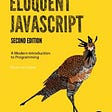# Introduction

In this post, we will cover creating primitive streams, using Optional types with primitives, and summarizing statistics in a single pass.

# Primitive Streams

There are three types of primitive streams: IntStream (int, short, byte, and char), LongStream (long), and DoubleStream (double and float).

## Creating Primitive Streams

Creating primitive streams is the same as a regular Stream. In this example, we created an empty stream, a single element stream and a stream from an array with respectively.

`IntStream empty = IntStream.empty();IntStream IntStream<Integer> singleElement = IntStream.of(1);IntStream IntStream<Integer> arrayStream= IntStream.of(1,2,3);`

Let’s create an infinite primitive stream. As we generate an infinite stream, the program will not finish until we terminate it.

`IntStream randomNumbers = IntStream.generate (Math::random); IntStream iterateForever = IntStream.iterate(1, n -> n * 2);`

It is common to count the elements of the stream using the limit() function. This example prints out the numbers 1, 4, 9.

`IntStream iterateForever = IntStream.iterate(1, n -> n * 2); IntStream count = iterateForever.limit(3); count.forEach(System.out::println); // 1 4 9`

Another way to create a primitive stream is by range() and rangeClosed() function.

`IntStream range = IntStream.range(1, 5); range.forEach(System.out::println); // 1 2 3 4 IntStream rangeClosed = IntStream.rangeClosed(1, 5); rangeClosed.forEach(System.out::println); // 1 2 3 4 5`

The last way is mapping from another stream type. Note that

`Stream<String> objStream = Stream.of("komutan", "logar"); IntStream intStream = objStream.mapToInt(s -> s.length())`

## Optionals with Primitive Streams

Optional types for primitives are OptionalInt, OptionalDouble, and OptionalLong. We are going to cover the max, sum, average, getAsInt, getAsDouble, getAsLong, and orElseGet.

Get the maximum value of a stream with max()

The max() method returns an Optional value of an int, a double, or a long.

`IntStream streamIntMax = IntStream.of(10, 32, 13, 44);OptionalInt optionalInt = streamIntMax.max();int intValue = optionalInt.getAsInt();assertEquals(44, intValue); DoubleStream streamDoubleMax = DoubleStream.of(3, 5, 10);OptionalDouble optionalDouble = streamDoubleMax.max();double doubleValue = optionalDouble.getAsDouble();assertTrue(doubleValue == 10); LongStream streamLongMax = LongStream.of(2L, 5L, 10L);OptionalLong optionalLong = streamLongMax.max();long longValue = optionalLong.getAsLong();assertEquals(10L, longValue);`

Get the sum of elements in a stream with sum()

The sum() method returns the corresponding value of the stream, such as int, double, or long.

`IntStream streamIntSum = IntStream.of(10, 32, 13, 44);int sumInt = streamIntSum.sum();assertEquals(99, sumInt); DoubleStream streamDoubleSum = DoubleStream.of(3, 5, 10);double sumDouble = streamDoubleSum.sum();assertTrue(18.0 == sumDouble); LongStream streamLongSum = LongStream.of(2L, 5L, 10L);long sumLong = streamLongSum.sum();assertEquals(17L, sumLong);`

Get arithmetic mean of elements of a stream with average()

The average() method always returns OptionalDouble type for all types of primitive streams.

`IntStream streamIntAvg = IntStream.of(10, 32, 13, 44);OptionalDouble avgInt = streamIntAvg.average();avgInt.ifPresent(System.out::println);assertTrue(avgInt.isPresent());assertTrue(avgInt.getAsDouble() == 24.75); DoubleStream streamDoubleAvg = DoubleStream.of(3, 5, 10);OptionalDouble avgDouble = streamDoubleAvg.average();avgDouble.ifPresent(System.out::println);assertTrue(avgDouble.isPresent());assertTrue(avgDouble.getAsDouble() == 6.0); LongStream streamLongAvg = LongStream.of(2L, 9L, 10L);OptionalDouble avgLong = streamLongAvg.average();assertTrue(avgLong.isPresent());avgLong.ifPresent(System.out::println);assertTrue(avgLong.getAsDouble() == 7.0);`

Return an alternative value with orElseGet()

The orElseGet() method takes a Supplier. It returns the value if present, otherwise returns the result of the calling Supplier. The method signature is orElseGet(Supplier s).

`IntStream streamIntAvg = IntStream.of(10, 32, 13, 44); OptionalDouble avgInt = streamIntAvg.average(); System.out.println(avgInt.orElseGet(()->10.0));`

## Summarizing Statistics

summaryStatistics() method computes summary statistics, such as maximum, sum, and average of the stream elements, in a single pass. This is really useful as we can’t operate multiple terminal operations for the same stream.

`IntStream streamStatistics = IntStream.of(10, 32, 13, 44);IntSummaryStatistics stats = streamStatistics.summaryStatistics();assertEquals("IntSummaryStatistics{count=4, sum=99, min=10, average=24.750000, max=44}", stats.toString());assertEquals(99, stats.getSum());assertEquals(10, stats.getMin());assertTrue(24.750000 == stats.getAverage());assertEquals(44, stats.getMax());`

# Summary

In this section, we covered the three primitives IntStream, LongStream, and DoubleStream. We learned how to use Optional types with primitives, and summarizing statistics on a stream in a single pass. You can find the source code on GitHub.

Originally published at http://suleymanyildirim.org on March 5, 2020.

## More from Suleyman Yildirim

Software Engineer, Oracle Certified Java Programmer, http://suleymanyildirim.org/

Love podcasts or audiobooks? Learn on the go with our new app.

## How to use JSX in markdown documents## What Is the Best Book for Learning JavaScript?## The mysterious “this” in JavaScript## Templating Projects On .NET Core## Build a Flexible React Icon Component## Mini-Project: Refactored Checkbook Balancer## Suleyman Yildirim

Software Engineer, Oracle Certified Java Programmer, http://suleymanyildirim.org/

## Looping in Java## File handling in java## What is java?## what is Garbage Collector, Stack ,Heap in JAVA?